Goseeko blog# What is FFT?

Certain algorithms permit implementations of Discrete Fourier transform with considerable savings in computation time. These algorithms are known as Fast Fourier Transform. Also known as FFT.

FFT algorithms are based on the fundamental principle of decomposing the computation of DFT of sequence length into successively smaller discrete Fourier transforms.

They are basically two classes of FFT algorithms

• Decimation in time
• Decimation in frequency

## Decimation-in-time algorithm FFT

This algorithm is known as Radix-2 DIT-FFT algorithm which means the number of output points N can be expressed as a power of 2 that is N = 2M  where M is an integer.

Let x(n) be a sequence where N is assumed to be a power of 2. Decimate or break this sequence into two sequences of length N/2 where one sequence consists of even-indexed values of x(n) and the other of odd-indexed values of x(n).

xe(n)  = x(2n)   n= 0,1,……….. N/2-1

xo(n) = x(2n+1)  n= 0,1,……….. N/2 -1

The N-point DFT of x(n) can be written as

Separating x(n) into even and odd indexed values of x(n) we obtain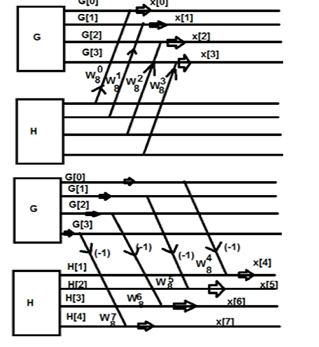W84 = -1

and W8 5 = – W 81

also W86 = – W82

W87 = – W83

G(0) – H(0) = x(4)

G(1)  – W 81 H(1) = x(5)

G(2) – W82 H(2) = x(6)

G(3) – W83  H(3) = x(7)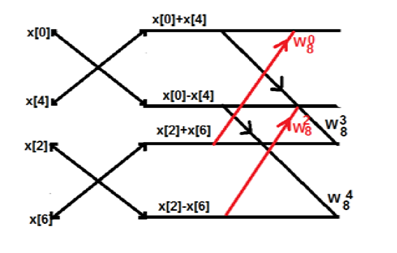Let  us consider one example now, to understand the concept.

Consider the sequence x[n]={ 2,1,-1,-3,0,1,2,1}. Calculate the FFT.

Arrange the sequence as x(0) x(4) x(2) x(6) x(1) x(5) x(3) x(7)

Since N=8 find the values W8 0 to W 87

Apply the butterfly diagram to obtain the  values.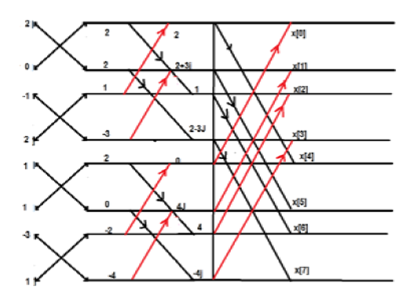## Decimation in Frequency

Apart from time sequence, we can represent an N-point sequence in frequency. Let us take a four-point sequence to understand it better.

Let the sequence be x,x,x,x………..x

Mathematically, this sequence will be as follows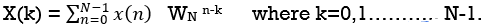Now let us make one group of sequence numbers 0 to 3 and another group of sequence 4 to 7. Now, mathematically this will be as follows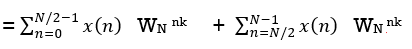Let us replace n by r, where r = 0, 1 , 2….N/2−1.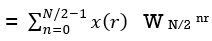We take the first four points  x,x,x,x initially, and try to represent them mathematically as follows –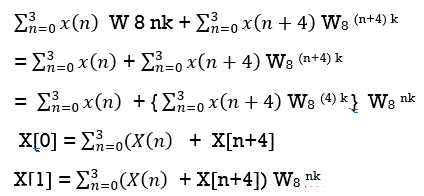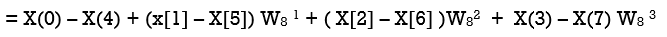Interested in learning about similar topics? Here are a few hand-picked blogs for you!